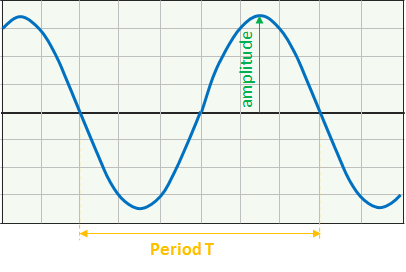### The Basic Quantities of AC waveform

An AC waveform is constantly changing its polarity every half cycle alternating between a positive maximum value and a negative maximum value. This signifies that the AC waveform is a time-dependent signal.AC Waveform Characteristics

Period (T)
Period time refers to the time in seconds that the waveform takes to repeat itself from start to finish, that means the time duration of one cycle. The best approach in measuring the period is to use successive crossings of the zero axis with a positive or negative slope.

Frequency (f)
Frequency refers to the number of times the waveform repeats itself within one second time period. It is commonly measured in cycles per second (1/sec or sec-1) and is expressed in units of Hertz (Hz). The relation between frequency and time period is:

f = 1 / T

Amplitude (A)
Amplitude refers to the magnitude of the signal waveform measured in volts or amps. It is the maximum value, positive or negative, that the waveform can attain.